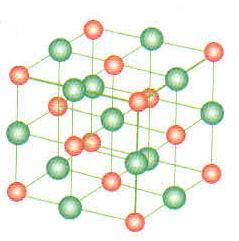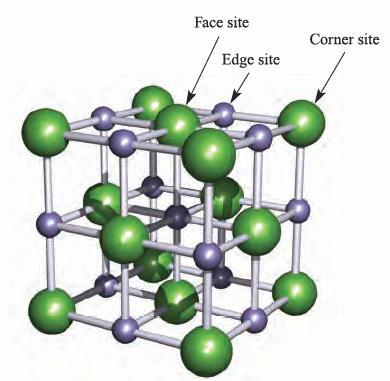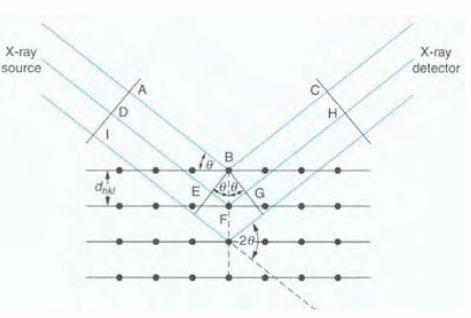### Lab Report on Qualitative and quantitative analysis of alkali halide mixture by powder X-ray diffraction

The lab report below was submitted as part of the coursework for CM3292 Advanced Experiements in Analytical and Physical Chemistry. Please do not plagiarise from it as plagiarism might land you into trouble with your university. Do note that my report is well-circulated online and many of my juniors have received soft copies of it. Hence, please exercise prudence while referring to it and, if necessary, cite this webpage.

1. Aim
This experiment uses X-ray powder diffraction technique to identify crystal structures, determine the structural parameters for alkali halides and quantitatively analyse the composition of alkali halide mixtures.

1. Results and calculations
Using the sample A1 of pure KCl for calculations, the following results were obtained:
 2θ / ° sin2θ h2 + k2 + l2 Integer Simple cubic plane 28.559 0.060837 1.000000 1 100 40.770 0.121331 1.994358 2 110 50.403 0.181308 2.980212 3 111 58.869 0.241502 3.969629 4 200 66.604 0.301458 4.955148 5 210 73.854 0.360957 5.933148 6 211

Given h2 + k2 + l2 = (4a22)sin2θ, and that λ = 1.5405, and that gradient = λ2 / 4a2= 0.0600,
Solving, a = 3.1445
For a simple cubic model, there is only 1 molecule of KCl per unit cell.
Density using obtained parameter = 1 ×74.556.022 × 1023 ×(3.1445 × 10-8)3 = 3.981g/cm3
Comparing this obtained density value to the macroscopic density of 1.984 g/cm3, the obtained value does not coincide. Therefore, KCl does not have a simple cubic structure.
Using the FCC model for KCl instead, the following results were obtained:
 2θ  / ° sin2θ h2 + k2 + l2 FCC integer FCC plane 28.559 0.060837 1.000000 4 200 40.770 0.121331 1.994358 8 220 50.403 0.181308 2.980212 12 222 58.869 0.241502 3.969629 16 400 66.604 0.301458 4.955148 20 420 73.854 0.360957 5.933148 24 422

Following similar calculations as done above for the simple cubic model,λ2 / 4a2= 0.0150, a = 6.289
For FCC model, there are 4 molecules per unit cell,
Density = 4 ×74.5516.022 × 1023 ×(6.289 × 10-8)3 = 1.9908 g / cm3
This calculated value using the FCC model is closer to the given value of 1.984 g/cm3. Hence, the Bravais lattice for KCl is face-centred cubic.
Given that the ionic radius of K+ ion is 1.34,Ionic radius of Cl- ion = 3.1445 - 1.34 =1.805
The experimental radius of Cl- is close to its reported value of 1.81 .
The following results were obtained for sample A5 of pure NaCl:
 2θ  / ° sin2θ h2 + k2 + l2 FCC integer FCC plane 27.535 0.056636 1.000000 3 111 32.059 0.076249 1.346307 4 200 45.583 0.150062 2.649607 8 220 56.626 0.224949 3.971860 12 222 66.355 0.299466 5.287578 16 400 75.404 0.373999 6.603593 20 420Gradient of the line = λ2 / 4a2 = 0.0186, a = 5.648
Density = 4 ×58.4436.022 × 1023 ×(5.648 × 10-8)3 = 2.044g / cm3
This calculated value compares favorably to the literature value of 2.165g / cm3, suggesting that NaCl has a face-centered cubic lattice.
From the earlier calculated radius of Cl- = 1.805,
Ionic radius of Na+ ion = (5.648/2) – 1.805 = 1.019The experimental radius of Na+ is close to its reported value of 1.020 , suggesting that the experiment is highly accurate. The Cl- ions are larger than the Na+ ions. The Cl- ions are represented by the larger spheres in the above diagram.
Qualitative analysis
 Mixtures of NaCl and KCl Mass / gram Weight / % (200) peak intensity (200) peak intensity % Sample NaCl KCl NaCl NaCl KCl NaCl KCl A1 0.0000 2.0002 0.00 0 1577 0.00 100.00 A2 0.5051 1.5001 25.19 4538 5827 43.78 56.22 A3 1.0004 1.0097 49.86 2785 1596 63.57 36.43 A4 1.5024 0.5008 75.00 3437 854 80.10 19.90 A5 2.0008 0.0000 100.00 12096 0 100.00 0.00 Unknown A 7973 6974 53.34 46.66

Peak intensity of NaCl in unknown sample = 57.37%
Using the graph, the weight percentage of NaCl in unknown sample = (57.37 – 10.17) / 0.946
= 49.89% NaCl by weight
Weight percentage of KCl in unknown sample = 100 – 49.89 = 50.11% KCl by weight
1. Discussion
In this experiment, Powder X-Ray Diffraction (XRD) is used to determine the crystallographic structures of different alkali halides. The crystalline powder of these samples will diffract incident X-ray beams if their crystallites are coincidentally oriented such that it obeys the Bragg equation.
Principles of XRD* Diagram showing X-ray diffraction by a plane 
From the right figure, the path difference of the X-ray beams is given by EF + FG. They are equidistant, and can be calculated by EF = FG = dhklsinθ. For constructive interference to occur, the waves must be at integer value difference from each other, and Bragg’s law can be written as nλ = 2 dhklsinθ. For a cubic unit cell system, dhkl2 =a2h2+ k2+ l2, and Bragg’s law can thus be rewritten as h2 + k2 + l2 = (4a22)sin2θ. In the machine used, the disc is gradually rotated over a range of angles to obtain the different diffraction patterns (bottom right diagram).* Diagram showing the operation by the machine in determining the angles 
In cubic systems, the first X-ray diffraction signal in the spectrum is due to the diffraction by the plane with the lowest Miller indices. For a cubic unit cell, the following are possible:
Simple cubic (100) plane, h2 + k2 + l2 = 1
BCC (110) plane, h2 + k2 + l2 = 2
FCC (111) plane, h2 + k2 + l2 = 3
As such, the value of sin2 q/ sin2 qmin has to be multiplied with the appropriate integer value to determine the Bravais lattice type.
The position of the atoms and the scattering factor affects the peak intensity. For KCl, the scattering factor is the same for the cations and anions. The following structure factors apply to FCC lattices:
Fhkl = 4(f+ + f-) for all even values of Miller indices
Fhkl = 4(f+ - f-) for all odd values of Miller indices
Fhkl = 0 for mix of odd and even Miller indices
where f+ and f- represent the scattering factor of the cations and anions respectively. It thus follows that the reflection intensity will only be recorded for even values of Miller indices since the odd ones will cancel out. This accounts for the systematic absence recorded where some reflections expected were not observed.
X-Ray Diffractograms of NaCl and KCl
The angles θ (given by the position of the lines) due to the same set of Miller planes are slightly larger in NaCl. This is because the NaCl unit cell is slightly smaller than that of KCl. The more striking difference is the absence of certain lines like (111), (311), (511) etc. in KCl although these lines are present in powder pattern of NaCl. From systematic absence rules for FCC crystals, we know that the lines with mixed indices should be absent for both NaCl and KCl. This is indeed so. Further, for NaCl it can be observed that the successive orders of (111) planes (these are 111, 222, 333, 444 etc.) are alternately weak and strong. For example, the reflection from (111) is weak and that from (222) is strong and so on. In the binary fcc compounds like NaCl or KCl, the (111) planes are alternately occupied by Na+ (or K+) and Cl ions. If the scattered waves from two or more such planes containing only sodium are in phase and intensify the reflection, the planes containing sodium will be out of phase with planes containing chlorine and will interfere destructively thus diminishing the intensity. This is the reason why (111) reflection in NaCl is weak and (222) reflection is strong. For KCl, the alternate (111) planes have potassium and chlorine. These two ions have the same number of electrons and identical scattering power. So far as X-ray is concerned, K+ and Cl are identical. Since the radiation scattered by these planes are out of phase, the reflected waves will get completely annihilated and the reflections from (111), (333) etc. will not be seen.

At the end of the XRD, it was observed that the disc A1 had some purple colouration and disc A5 had a yellowish brown colouration. This is due to phosphorescene. When the X-ray beams were incident on the compounds for a long period of time, they were excited to the triplet state from the singlet state despite this being a spin forbidden transition. Similarly, it would take a long period of time for the excited triplet state to lose energy to return to the ground singlet state. In the midst of doing so, visible light is given off, thus allowing us to observe colouration on the plates. Comparing with literature values, NaCl absorbs at about 450nm and KCl absorbs at about 550nm. Thus a complementary colour of yellow and purple were observed for NaCl and KCl respectively. The other discs did not have as distinct a colouration as A1 and A5, possibly due to the mixture of 2 different salts.
The experimental radius of Na+ (1.019) is close to its reported value of 1.02 . The experimental radius of Cl- (1.805) is close to its reported value of 1.81 , suggesting that the experiment is highly accurate.
Limitations of XRD
Initial calculations of h2 + k2 + l2 for KCl seemed to show that it has a simple cubic unit cell. This can be explained by the same number of electrons on the cation and anion, and the same ability to disperse the X-ray beams. Consequently, the X-ray beams were not able to differentiate between the two different elements and KCl appears to have a simple cubic lattice when it is actually a FCC lattice.
While X-ray diffraction is sufficient in determining the atomic positions and the Bravais lattice type, it has its limitations too. The bond length as determined by X-ray diffraction is distorted by the electron cloud, and will be shorter than actual. A more accurate alternative would be to employ neutron diffraction.
1. Conclusion
The results are summarised as follows:
 Salt Molecular weight (g/mol) Macroscopic Density (g/cm3) Bravais Lattice Type Unit Cell Dimension (Å) Crystallographic density (g/cm3) NaCl 58.443 2.165 Face-centered cubic 5.648 2.172 KCl 74.551 1.984 Face-centered cubic 6.289 1.991

The ionic radii of Na+ ion and Cl- ion are 1.019 and 1.805 respectively. The weight percentage of the unknown sample was determined to be 49.89% weight NaCl and 50.11% weight KCl.
1. Exercise
1. In both KCl and NaCl, the Cl- ions can be viewed as lying on the (111) plane. In between these (111) planes, layers of the cations (K+ or Na+) lie. The reflection of the X-ray beams from Cl- would be completely out of phase with the reflection by Na+. As such, destructive interference would take place.
For KCl, both K+ and Cl- ions have 18 electrons. They are isoelectronic. As such, they disperse X-ray beams to a similar extent. Destructive interference would then occur, resulting in the cancellation of the reflections of the X-ray beams. Thus, the (111) reflection appears to be absent.
For NaCl and KBr, the cations have fewer electrons than the anions. The abilities of the ions to scatter X-rays are different. As such, their reflections of X-rays will only partially cancel out each other and give a weak peak.  Specifically, the (111) reflection in NaCl corresponds to one wavelength of path difference between the neighbouring (111) planes. 

1. Given the values for tungsten metal, the following results were obtained:
 2θ  / ° sin2θ h2 + k2 + l2 Simple Cubic integer BCC integer 40.262 0.118451 1 1 2 58.251 0.236900 1.999983115 2 4 73.184 0.355350 2.999974673 3 6 86.996 0.473797 3.999940904 4 8 100.632 0.592250 4.999957788 5 10 114.923 0.710700 5.999949346 6 12

Using the simple cubic model and the equation h2 + k2 + l2 = (4a22)sin2θ,
Gradient = λ2 / 4a2 = 0.1184, a = 2.238
Density = 1 ×183.846.022 × 1023 ×(2.238 × 10-8)3 = 27.234g / cm3
This calculated value differs greatly from the literature density value. Hence, the Bravais lattice of tungsten is not simple cubic.
Gradient = λ2 / 4a2 = 0.0592, a = 3.166
Density = 2 ×183.846.022 × 1023 ×(3.166 × 10-8)3 = 19.240g / cm3
This calculated value is close to the literature value for density of tungsten. Therefore, it can be determined that tungsten has a body centred cubic Bravais lattice and a unit cell length of 3.166.
1. References
1. Shriver & Atkins, Inorganic Chemistry, 4th edition, Oxford.
2. Jim Clark, Atomic and Ionic Radius, 2012.
3. Lesley E. Smart, Elaine A. Moore, Solid State Chemistry, 3rd edition, CRC.
4. University of Gaziantep, X-Ray Diffraction in Crystal, 2011.
5. Skoog, D. A., West, D. M., Holler, F. J., & Crouch, S. R. . Fundamaentals of Analytical Chemistry, 2004.

1.